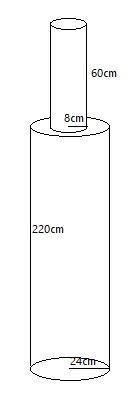Courses
Courses for Kids
Free study material
Free LIVE classes
MoreLIVE
Join Vedantu’s FREE Mastercalss

# A solid iron pole consists of a cylinder of height $220cm$ and base diameter $24cm$ which is surmounted by another cylinder of height $60cm$ and radius $8cm$. Find the mass of the pole, given that $1c{{m}^{3}}$ of iron has approximately $8g$ mass. (Use $\pi =3.14$).Verified
362.1k+ views
Hint: Given structure might seem quite complex but considering it by parts, we can easily solve it. Like this pole is a combination of two cylinders placed on top of one another. Calculating required dimensions one by one for each cylinder we can calculate values for the whole pole.

Here as per the diagram we can say that, there are two cylinders of different dimensions but of the same material.
Considering cylinder1 as bottom cylinder and cylinder2 as top cylinder, we can easily calculate the required dimensions for the complete pole.
Now, the dimensions of cylinder1 are: Diameter = ${{d}_{1}}=24cm$, height = ${{h}_{1}}=220cm$.
As, radius = $\dfrac{\text{Diameter}}{2}$
$\therefore {{r}_{1}}=\dfrac{{{d}_{1}}}{2}=\dfrac{24}{2}=12cm$.
While dimensions for cylinder2 are: Radius = ${{r}_{2}}=8cm$, height = ${{h}_{2}}=60cm$.As we have to find the mass of the pole, we first have to calculate the volume of the whole structure, which is the sum of volumes of cylinder1 and cylinder2.
And we know that, the volume of a cylinder of height $h$ and radius $r$ is $V=\pi {{r}^{2}}h$.
On applying given dimensions of cylinders, we have
Volume of cylinder1 =${{V}_{1}}$ , i.e.,
\begin{align} & {{V}_{1}}=\pi {{r}_{1}}^{2}{{h}_{1}} \\ & =\pi {{\left( 12 \right)}^{2}}\left( 220 \right) \\ & =\pi \left( 144 \right)\left( 220 \right) \\ & =3.14\times 144\times 220 \\ & =99475.2c{{m}^{3}}...\text{ }\left( 1 \right) \end{align}
Similarly, for cylinder2 we have
Volume of cylinder2 = ${{V}_{2}}$, i.e.,
\begin{align} & {{V}_{2}}=\pi {{r}_{2}}^{2}{{h}_{2}} \\ & =\pi {{\left( 8 \right)}^{2}}\left( 60 \right) \\ & =\pi \left( 64 \right)\left( 60 \right) \\ & =3.14\times 64\times 60 \\ & =12057.6c{{m}^{3}}...\text{ }\left( 2 \right) \end{align}
From the given condition, total volume of pole = volume of cylinder1 + volume of cylinder2, i.e.,
$V={{V}_{1}}+{{V}_{2}}$
Substituting the values from equation (1) and (2), we get
\begin{align} & V=99475.2+12057.6 \\ & =111532.8c{{m}^{3}}...\text{ }\left( 3 \right) \end{align}
Now, to calculate the mass of pole we have to use mass-density formula, i.e.,
$Density=\dfrac{Mass}{Volume}...\text{ }\left( 4 \right)$
And from given values, we have
$Density=\rho =\dfrac{8g}{1c{{m}^{3}}}=8gm/c{{m}^{3}}$.
Substituting values from equation (3) in equation (4), we get
\begin{align} & Density=\dfrac{Mass}{Volume} \\ & Mass=Density\times Volume \\ \end{align}
$m=V\times \rho =111532.8\times 8=892262.4gm$
Or, $m=892.262kg$
Hence, Mass of the pole is $m=892.262kg$.

Note: Students might make a mistake in finding the volume of pole, at first. They might not consider it part by part to calculate its volume. It becomes very easy to calculate dimensions if a structure is broken into basic models.
Last updated date: 27th Sep 2023
Total views: 362.1k
Views today: 9.62k